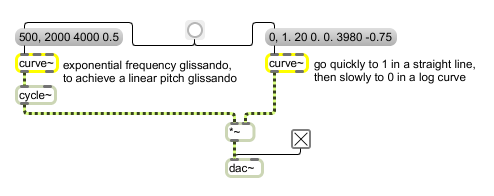curve~

Exponential ramp generator

Description

curve~ is similar to line~. It produces a signal that goes from an initial to target value over a specified time.
This object uses the Max time format syntax; envelope times can be either single valued fixed or tempo-relative.
However, it produces non-linear ramps using a piecewise approximation of an exponential function. You specify the parameter to this exponential function in the curve~ object's right inlet. Values from 0 to 1 produce an "exponential" curve when increasing in value and values from -1 to 0 produce a "logarithmic" curve. The closer to 0 the curve parameter is, the closer the curve is to a straight line, and the farther away the parameter is from 0, the steeper the curve. curve~ can also accept a list of up to two or three arguments (the previous curve parameter is used if there are two arguments), or a list with two or more value, time, parameter triples. The curve~ is limited to 42 such triples in a single list. The object's approximation of the exponential becomes better when the vector size is smaller, but the object also becomes more computationally expensive.

Arguments

Name Type Opt Description
initial-value and curve-parameter (floats or ints) list opt The first argument sets an initial value for the signal output. The second argument sets the initial curve parameter. The default values for the initial signal output and curve parameter are 0.

Messages

 int target-value [int] In left inlet: The number is the target value, to be arrived at in the time specified by the number in the middle inlet. If no time has been specified since the last target value, the time is considered to be 0 and the output signal jumps immediately to the target value (ints are converted to floats). float target-value [float] In left inlet: The number is the target value, to be arrived at in the time specified by the number in the middle inlet. If no time has been specified since the last target value, the time is considered to be 0 and the output signal jumps immediately to the target value. list target-value ramp-time (milliseconds) and curve-parameter (between -1 and 1) [list] In left inlet: The first number specifies a target value; the second number specifies an amount of time, in milliseconds, to arrive at that value; and the optional third number specifies a curve parameter, for which values from 0 to 1 produce an exponential curve and values from -1 to 0 produce a logarithmic curve. The closer to 0 the curve parameter is, the more the curve resembles a straight line, and the farther away the parameter is from 0, the more the curve resembles a step. In the specified amount of time, curve~ generates an exponential ramp signal from the currently stored value to the target value. In middle inlet: A list may be used to specify time in one of the Max time formats. anything object-parameters [list] Equivalent to list. factor non-linearizing-factor [float] The word factor followed by a float will adjust the non-linear shaping parameters of curve~. pause In left inlet: Pauses the internal exponential ramp but does not change the target value nor clear pending target-time-parameter triples. curve~ will continue outputting whatever value was its current value when the pause message was received, until either it receives a resume message or until a new ramp is input. stop In left inlet: Stops the internal exponential ramp and clears pending target-time parameter triples. curve~ will continue outputting whatever value was its current value when the stop message was received, resetting its target value to that value. resume In left inlet: Resumes the internal exponential ramp and subsequent pending target-time pairs if the curve~ object was paused as a result of the pause message.

Output

bang: Out right outlet: When curve~ has finished generating all of its ramps, bang is sent out.
signal: Out left outlet: The current target value, or an exponential curve moving toward the target value according to the most recently received target value, transition time, and curve parameter.

ExamplesCurved ramps used as control signals for frequency and amplitude